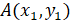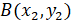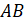### CO-ORDINATE GEOMETERY 2

Mathematics SSS 3 First Term

WEEK 10

CO-ORDINATE GEOMETERY 2

Performance Objectives

Student should be able to:

1. Calculate the gradient of a straight line and y intercept.
2. Determine the equation of a straight line and the angle between two intersecting lines.
3. Know the conditions for parallel line and perpendicular and how to apply them.

Content

In mathematics, the term gradient or slope is often used to describe how steeply a straight line lies. Given two pointsandto form a line,  we define the gradient of the lineView More